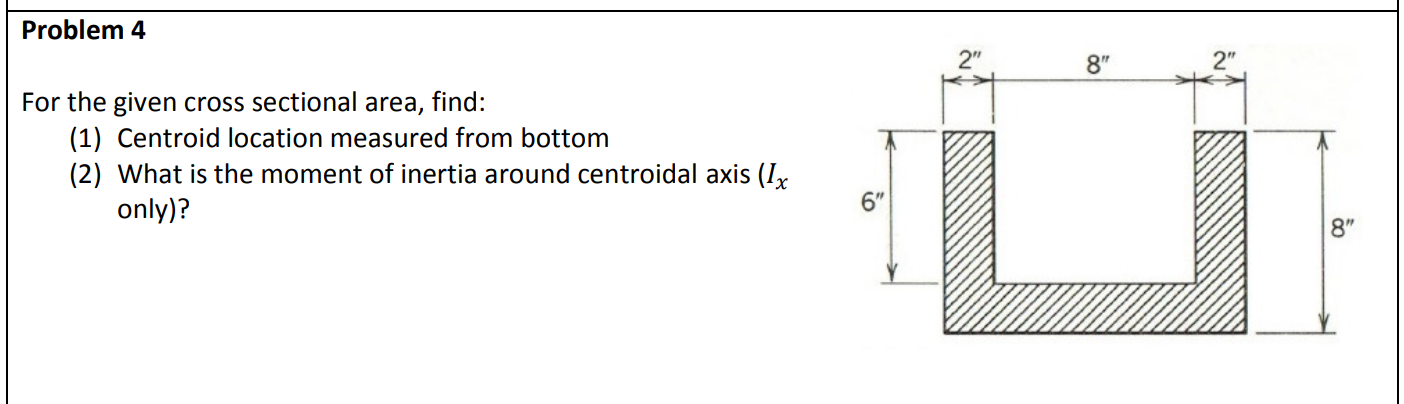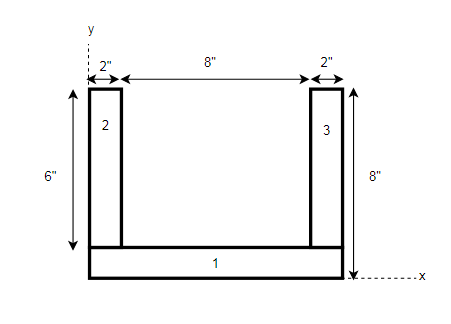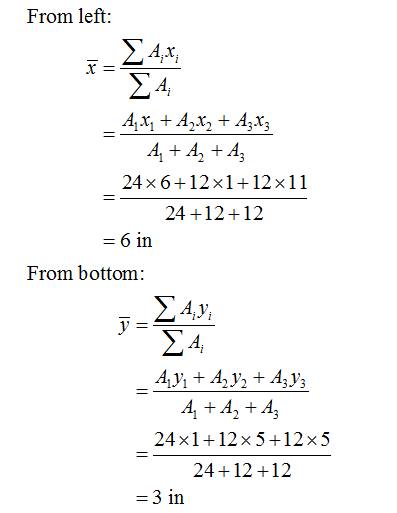# Problem 42"2"8"For the given cross sectionalarea,find:(1) Centroid location measured from bottom(2) What is the moment of inertia around centroidal axis (I,6"only)?8"

Question
87 viewshelp_outlineImage TranscriptioncloseProblem 4 2" 2" 8" For the given cross sectional area, find: (1) Centroid location measured from bottom (2) What is the moment of inertia around centroidal axis (I, 6" only)? 8" fullscreen
check_circle

Step 1

Divide the given member into three different rectangular section:Step 2

Calculate the individual area and the distance of the CG from the x and y-axis of the rectangular sections.

Rectangle 1:

A1 = 12 x 2 = 24 in2

x1 = 12/2 = 6 in.

y1 = 2/2 = 1 in.

Rectangle 2:

A2 = 2 x 6 = 12 in2

x2 = 2/2 = 1 in.

y2 = 2 + 6/2 = 5 in.

Rectangle 3:

A3 = 2 x 6 = 12 in2

x3 = 2 + 8 + 2/2 = 11 in.

y3 = 2 + 6/2 = 5 in.

Step 3

Calculating the centroid position from the left and from the bottom position:...

### Want to see the full answer?

See Solution

#### Want to see this answer and more?

Solutions are written by subject experts who are available 24/7. Questions are typically answered within 1 hour.*

See Solution
*Response times may vary by subject and question.
Tagged in